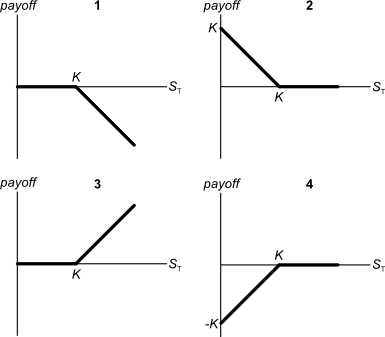# Fight Finance

#### CoursesTagsRandomAllRecentScores

A firm can issue 3 year annual coupon bonds at a yield of 10% pa and a coupon rate of 8% pa.

The beta of its levered equity is 2. The market's expected return is 10% pa and 3 year government bonds yield 6% pa with a coupon rate of 4% pa.

The market value of equity is $1 million and the market value of debt is$1 million. The corporate tax rate is 30%.

What is the firm's after-tax WACC? Assume a classical tax system.

Your friend just bought a house for $400,000. He financed it using a$320,000 mortgage loan and a deposit of $80,000. In the context of residential housing and mortgages, the 'equity' tied up in the value of a person's house is the value of the house less the value of the mortgage. So the initial equity your friend has in his house is$80,000. Let this amount be E, let the value of the mortgage be D and the value of the house be V. So $V=D+E$.

If house prices suddenly fall by 10%, what would be your friend's percentage change in equity (E)? Assume that the value of the mortgage is unchanged and that no income (rent) was received from the house during the short time over which house prices fell.

Remember:

$$r_{0\rightarrow1}=\frac{p_1-p_0+c_1}{p_0}$$

where $r_{0-1}$ is the return (percentage change) of an asset with price $p_0$ initially, $p_1$ one period later, and paying a cash flow of $c_1$ at time $t=1$.

A company runs a number of slaughterhouses which supply hamburger meat to McDonalds. The company is afraid that live cattle prices will increase over the next year, even though there is widespread belief in the market that they will be stable. What can the company do to hedge against the risk of increasing live cattle prices? Which statement(s) are correct?

(i) buy call options on live cattle.

(ii) buy put options on live cattle.

(iii) sell call options on live cattle.

Select the most correct response:

Below are 4 option graphs. Note that the y-axis is payoff at maturity (T). What options do they depict? List them in the order that they are numberedA stock was bought for $8 and paid a dividend of$0.50 one year later (at t=1 year). Just after the dividend was paid, the stock price was $7 (at t=1 year). What were the total, capital and dividend returns given as effective annual rates? The choices are given in the same order: $r_\text{total}$, $r_\text{capital}$, $r_\text{dividend}$. You want to buy an apartment priced at$500,000. You have saved a deposit of $50,000. The bank has agreed to lend you the$450,000 as a fully amortising loan with a term of 30 years. The interest rate is 6% pa and is not expected to change. What will be your monthly payments?

A share was bought for $30 (at t=0) and paid its annual dividend of$6 one year later (at t=1).

Just after the dividend was paid, the share price fell to $27 (at t=1). What were the total, capital and income returns given as effective annual rates? The choices are given in the same order: $r_\text{total}$ , $r_\text{capital}$ , $r_\text{dividend}$. A young lady is trying to decide if she should attend university. Her friends say that she should go to university because she is more likely to meet a clever young man than if she begins full time work straight away. What's the correct way to classify this item from a capital budgeting perspective when trying to find the Net Present Value of going to university rather than working? The opportunity to meet a desirable future spouse should be classified as: Which of the below formulas gives the profit $(\pi)$ from being short a put option? Let the underlying asset price at maturity be $S_T$, the exercise price be $X_T$ and the option price be $f_{LP,0}$. Note that $S_T$, $X_T$ and $f_{LP,0}$ are all positive numbers. A company advertises an investment costing$1,000 which they say is under priced. They say that it has an expected total return of 15% pa, but a required return of only 10% pa. Of the 15% pa total expected return, the dividend yield is expected to be 4% pa and the capital yield 11% pa. Assume that the company's statements are correct.

What is the NPV of buying the investment if the 15% total return lasts for the next 100 years (t=0 to 100), then reverts to 10% after that time? Also, what is the NPV of the investment if the 15% return lasts forever?

In both cases, assume that the required return of 10% remains constant, the dividends can only be re-invested at 10% pa and all returns are given as effective annual rates. The answer choices below are given in the same order (15% for 100 years, and 15% forever):